# A 20–lb force is applied to die control rod AB as shown. Knowing that the length of the rod is 9 in. and that α = 25°, determine the moment of the force about point B by resolving the force into horizontal and vertical components.

Question-AnswerCategory: Engineering MechanicsA 20–lb force is applied to die control rod AB as shown. Knowing that the length of the rod is 9 in. and that α = 25°, determine the moment of the force about point B by resolving the force into horizontal and vertical components.

A 20–lb force is applied to die control rod AB  as shown. Knowing that the length of the rod is 9 in. and that α = 25°, determine the moment of the force about point B by   resolving the force into horizontal and vertical components.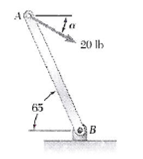Step: 1

Draw the free body diagram of the whole beam: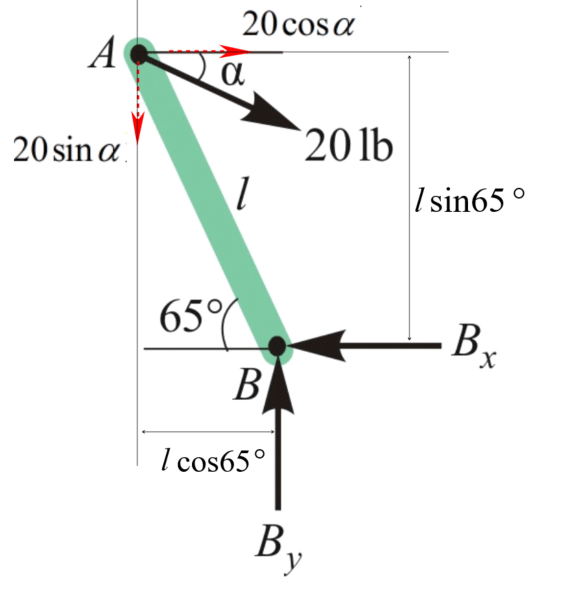Step: 2

Calculate the horizontal and vertical components of 20 lb force.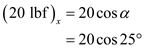Calculate the moment of 20 lb force about B.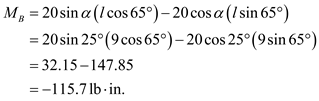Therefore, the moment of force about B,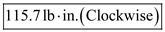.